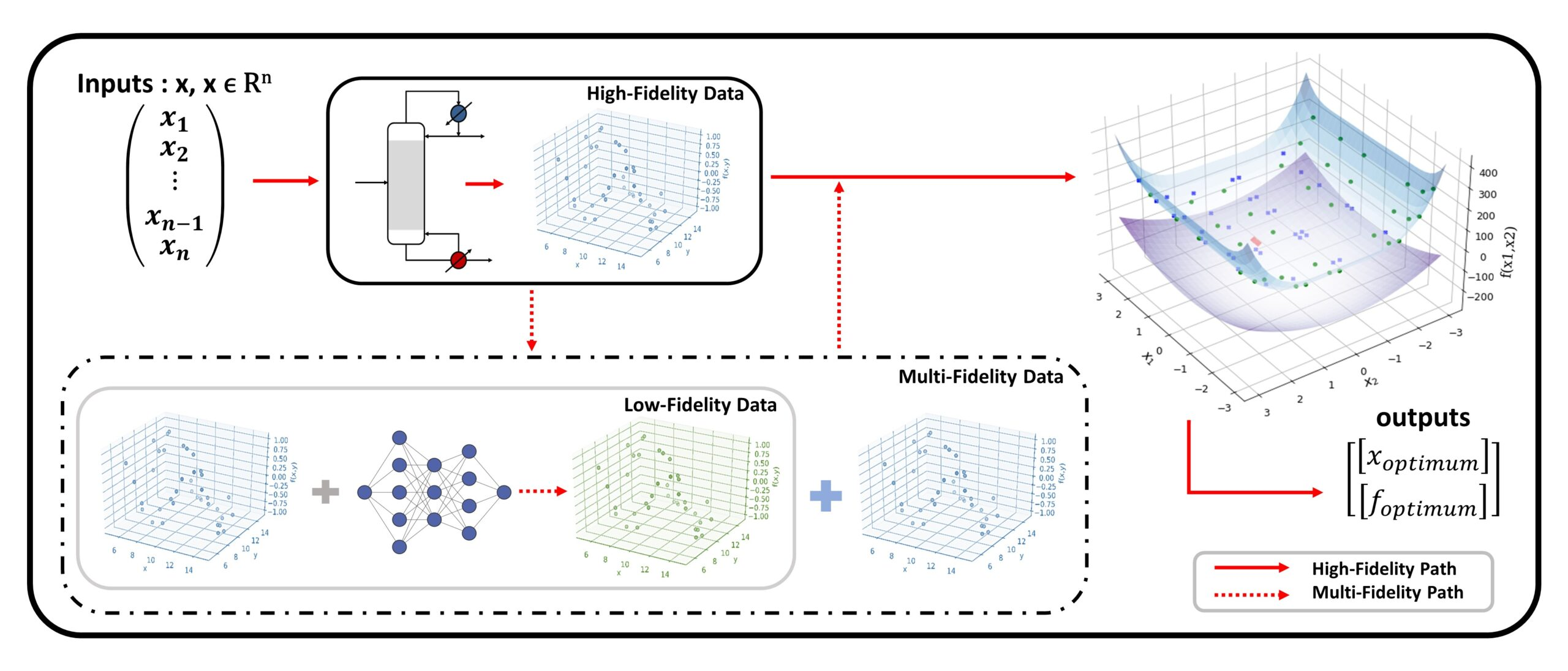Data-driven Spatial Branch-and-bound Algorithm for Box-constrained Simulation-based Optimization

Journal of Global Optimization 2021The ability to use complex computer simulations in quantitative analysis and decision-making is highly desired in science and engineering, at the same rate as computation capabilities and first-principle knowledge advance. Due to the complexity of simulation models, direct embedding of equation-based optimization solvers may be impractical and data-driven optimization techniques are often needed. In this work, we present a novel data-driven spatial branch-and-bound algorithm for simulation-based optimization problems with box constraints, aiming for consistent globally convergent solutions. The main contribution of this paper is the introduction of the concept data-driven convex underestimators of data and surrogate functions, which are employed within a spatial branch-and-bound algorithm. The algorithm is showcased by an illustrative example and is then extensively studied via computational experiments on a large set of benchmark problems.